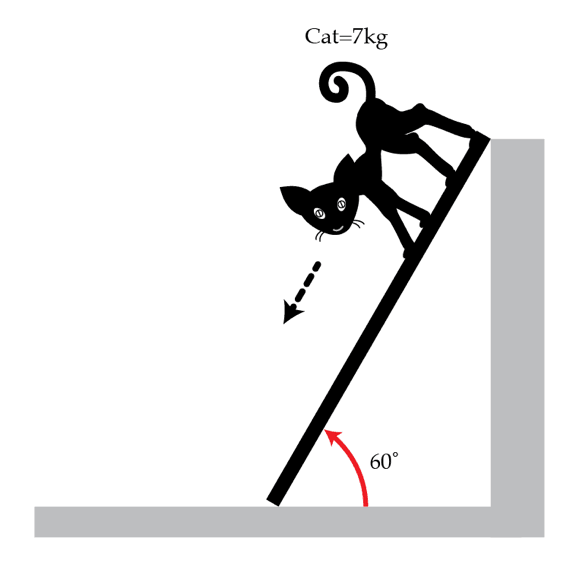# Ingenious Cat

A cat of mass $M=7~\text{kg}$ decides to descend down a light inclined plank of length $L=6~\mbox{m}$ which makes an angle of $\alpha=60^{\circ}$ with the horizontal, as shown in the figure below. The friction between the plank and the surfaces (the wall and the floor) can be neglected. The cat knows that walking on the plank may be dangerous. Nevertheless, the cat figures out a way to descend so that the plank remains at rest at all times. How long in seconds does it take the cat to reach the floor? The initial velocity of the cat is zero. You may ignore the mass of the plank. The cat remains in contact with the plank until he reaches the floor.Details and assumptions

$g=9.8~\text{m}/\text{s}^2$

×

Problem Loading...

Note Loading...

Set Loading...